﻿ 基于混沌时间序列的潜油柱塞泵动液面预测研究

# 基于混沌时间序列的潜油柱塞泵动液面预测研究Study of Forecasting Producing Fluid Level of Submersible Reciprocating Pump on the Basis of Chaotic Time Series

Abstract: Relying on the embedded computer system and automatic control technology, the submersible plunger pump is widely used in crude oil production, which improves the efficiency of crude oil extraction greatly. The core of the submersible plunger pump is the submersible linear motor which is located underground. The operation state, such as the impulse and the stroke, of the motor is controlled by computer on the ground, which can adapt the computer to the state of the oil wells to achieve higher efficiency and save more energy in the production of crude oil. Currently, the impulse control of the submersible plunger pump is based on the prediction and calculation of the time series of dynamic liquid surface. Our paper presents a method for predicting dynamic liquid surface on the basis of chaotic time series and analyzes its advantages compared with the linear prediction method. By this way, the dynamic liquid surface prediction method can be obtained to optimally control the submersible plunger pump impulse. Our research provides the theoretical and practical basis for the computer control of the submersible plunger pump.

1. 引言

2. 潜油柱塞泵原理及结构Figure 1. Structure diagram of submersible reciprocating pump

3. 动液面时间序列的特性分析

3.1. 动液面数据的采集

3.2. 动液面数据的预处理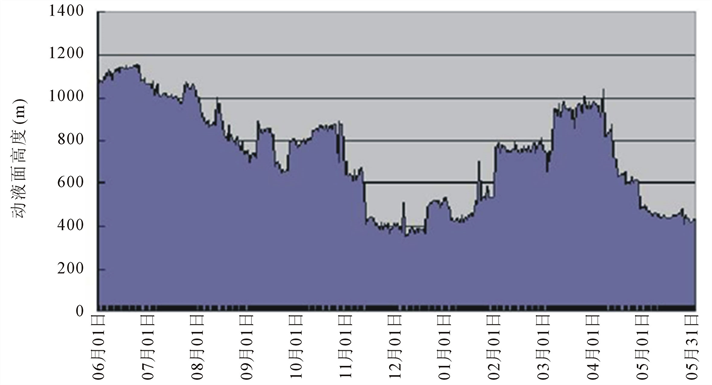Figure 2. Producing fluid level of oil well 54-12

3.3. 动液面时间序列混沌特性的识别

3.3.1. 饱和关联维数法

3.3.2. 最大Lyapunov指数法

Lyapunov指数是一个定量描述混沌运动对初始条件敏感度的量。混沌运动具有对其初始条件极为敏感的特征，即便轨道是由非常靠近的初始值形成的，也会随着时间快速分离。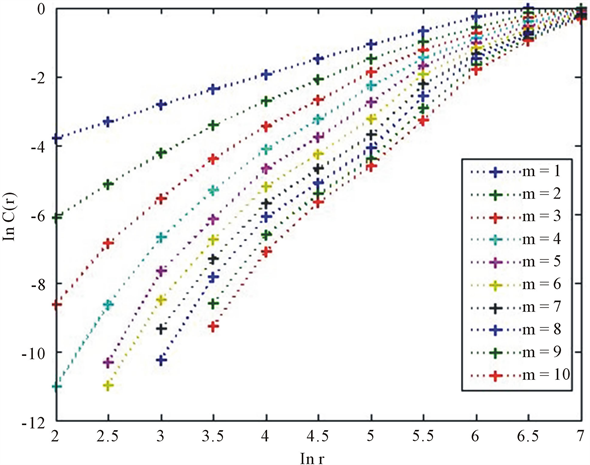Figure 3. The $\mathrm{ln}C\left(r\right)$ versus $\mathrm{ln}\left(r\right)$ plots for producing fluid level time series

$\epsilon {\text{e}}^{n\lambda \left({x}_{0}\right)}=|{F}^{n}\left({x}_{0}+\epsilon \right)-{F}^{n}\left({x}_{0}\right)|$ (1)

$\lambda \left({x}_{0}\right)=\underset{n\to \infty }{\mathrm{lim}}\underset{\epsilon \to 0}{\mathrm{lim}}\frac{1}{n}\mathrm{ln}|\frac{{F}^{n}\left({x}_{0}+\epsilon \right)-{F}^{n}\left({x}_{0}\right)}{\epsilon }|=\underset{n\to \infty }{\mathrm{lim}}\frac{1}{n}\mathrm{ln}{|\frac{\text{d}{F}^{n}\left(x\right)}{\text{d}x}|}_{x={x}_{0}}$ (2)

$\lambda =\underset{n\to \infty }{\mathrm{lim}}\frac{1}{n}\underset{i=0}{\overset{n-1}{\sum }}\mathrm{ln}{|\frac{\text{d}{F}^{n}\left({x}_{i}\right)}{\text{d}x}|}_{x={x}_{i}}$ (3)

3.4. 动液面时间序列最大预测时间

$\delta x\left(t\right)=\delta x\left(0\right){\text{e}}^{{\lambda }_{1}t}$ (4)

t为演化时间，当 $\frac{\delta x\left(t\right)}{\delta x\left(0\right)}$ 超出某个阈值c的时候，我们就认为轨道发散。这时的系统运动是不可预测的，而演化过程中的这个时间称为临界点时间 ${t}_{0}$ 。则：

$c=\frac{\delta x\left(t\right)}{\delta x\left(0\right)}={\text{e}}^{{\lambda }_{1}{t}_{0}}$ (5)

${t}_{0}=\frac{1}{{\lambda }_{1}}\mathrm{ln}c$ (6)Table 1. Largest Lyapunov exponent of producing fluid level time series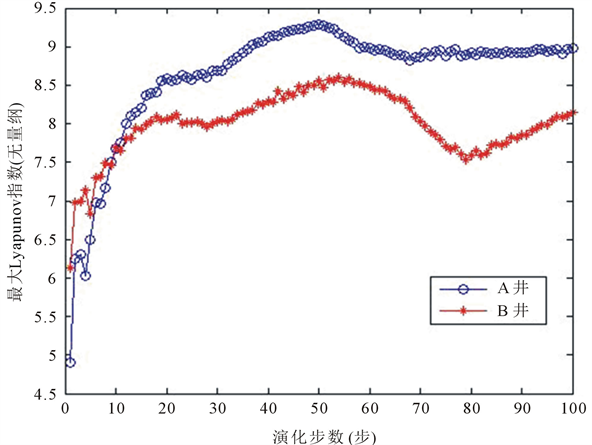Figure 4. Largest Lyapunov exponent of producing fluid level time series (i = 100)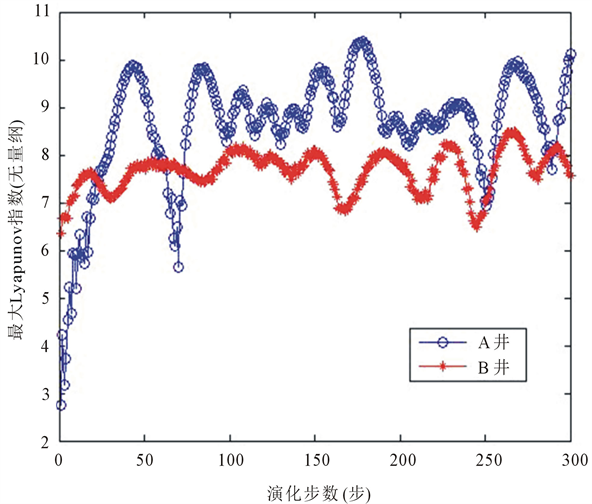Figure 5. Largest Lyapunov exponent of producing fluid level time series (i = 300)

${t}_{0}~\frac{1}{{\lambda }_{1}}$ (7)

1) A井动液面时间序列的可预测最大时间 ${t}_{0}$ 为1/0.1305~1/0.2318。所以，A井的动液面序列可预测最大时间大概为4天。

2) B井动液面时间序列的可预测最大时间 ${t}_{0}$ 为1/0.0457~1/0.319。所以，B井的动液面序列可预测最大时间大概是31天。

4. 动液面时间序列的预测

4.1. ARMA动液面线性预测模型

$\text{BIC}=-2\mathrm{ln}\left(L\right)+\left(p+q+1\right)\mathrm{ln}\left(n\right)$ (8)

n——一步预测时的残差个数。

$L\left({x}_{1},{x}_{2},\cdots ,{x}_{n},\theta \right)=f\left({x}_{1},\theta \right)f\left({x}_{2},\theta \right)\cdots f\left({x}_{n},\theta \right)$ (9)

$L\left({x}_{1},{x}_{2},\cdots ,{x}_{n},\stackrel{^}{\theta }\right)=\underset{\theta \in \Theta }{\mathrm{max}}\left\{f\left({x}_{1},\theta \right)f\left({x}_{2},\theta \right)\cdots f\left({x}_{n},\theta \right)\right\}$ (10)

$\stackrel{^}{\theta }=g\left({x}_{1},{x}_{2},\cdots ,{x}_{n}\right)$ 就是 $\theta$ 的极大似然估计值。

BIC最小值为7.6484，对应各参量， $p=6$$q=4$${\varphi }_{1}=-\text{0}\text{.7464}$${\varphi }_{2}=-\text{0}\text{.3726}$${\varphi }_{3}=-\text{0}\text{.4819}$${\varphi }_{4}=-\text{1}\text{.032}$${\varphi }_{5}=-\text{0}\text{.2172}$${\varphi }_{6}=\text{0}\text{.04302}$${\theta }_{1}=\text{0}\text{.499}$${\theta }_{2}=\text{0}\text{.215}$${\theta }_{3}=\text{0}\text{.3904}$${\theta }_{4}=\text{0}\text{.9314}$ ，不计白噪声项，油井动液面ARMA模型：

$\begin{array}{c}{X}_{t}=-\text{0}\text{.7464}{X}_{t-1}-\text{0}\text{.3726}{X}_{t-2}-\text{0}\text{.4819}{X}_{t-3}-\text{1}\text{.032}{X}_{t-4}-\text{0}\text{.2172}{X}_{t-5}\\ \text{\hspace{0.17em}}\text{\hspace{0.17em}}+\text{0}\text{.04302}{X}_{t-6}+\text{0}\text{.499}{\epsilon }_{t-1}+\text{0}\text{.215}{\epsilon }_{t-2}+\text{0}\text{.3904}{\epsilon }_{t-3}+\text{0}\text{.9314}{\epsilon }_{t-4}\end{array}$ (11)

4.2. 最大Lyapunov指数预测

Lyapunov指数是一个描述系统轨道指数发散程度的量化值，正是这个特性使它成为混沌时间序列的重要预测参数。令 ${Y}_{m}$ 表示预测的中心点。在构造的相空间中， ${Y}_{k}$${Y}_{m}$ 的最近邻点，这个距离记为 ${d}_{m}\left(0\right)$${\lambda }_{1}$ 为最大Lyapunov指数，则有：

$\left\{\begin{array}{l}{d}_{m}\left(0\right)=\underset{j}{\mathrm{min}}‖{Y}_{m}-{Y}_{j}‖=‖{Y}_{m}-{Y}_{k}‖\\ ‖{Y}_{m}-{Y}_{m+1}‖=‖{Y}_{k}-{Y}_{k+1}‖{\text{e}}^{{\lambda }_{1}}\end{array}$ (12)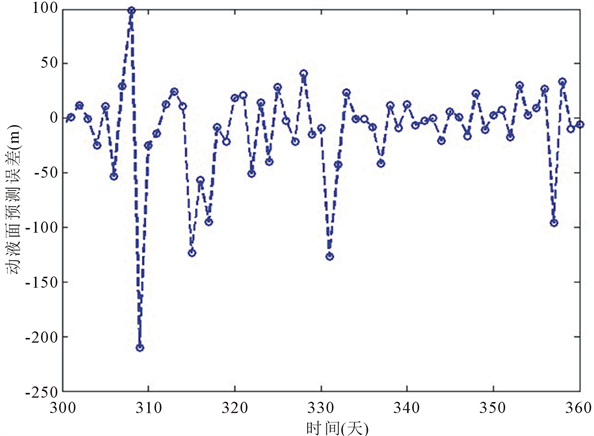Figure 6. Error curve of forecasting value for producing fluid level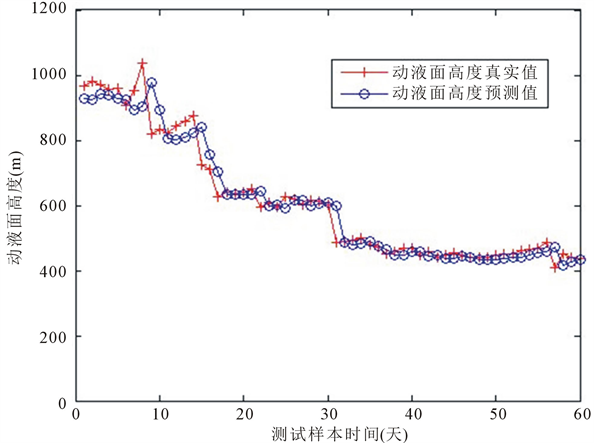Figure 7. Curves for producing fluid level forecasting

4.3. 预测误差分析

1) 平均相对误差：

$\text{MAPE}=\frac{1}{n}\underset{i=1}{\overset{n}{\sum }}|\left({y}_{d}\left(i\right)-{y}_{t}\left(i\right)\right)/{y}_{t}\left(i\right)|×100%$ (13)

2) 均方根误差：

$\text{RMSE}=\sqrt{\frac{1}{n}\underset{i=1}{\overset{n}{\sum }}{\left[{y}_{d}\left(i\right)-{y}_{t}\left(i\right)\right]}^{2}}$ (14)Table 2. Errors analyze for producing fluid level forecasting

3) 平方和相对误差：

$\text{PERR}=\underset{i=1}{\overset{n}{\sum }}{\left[{y}_{t}\left(i\right)-{y}_{d}\left(i\right)\right]}^{2}/\underset{i=1}{\overset{n}{\sum }}{y}_{t}^{2}\left(i\right)$ (15)

4) 相对误差在10%以内的后验概率，即 $\text{|}e\text{|}\le \text{10}%$ 的后验概率：

${P}_{\text{0}\text{.1}}=\frac{1}{n}\underset{i=1}{\overset{n}{\sum }}I\left\{|\left({y}_{d}\left(i\right)-{y}_{t}\left(i\right)\right)/{y}_{t}\left(i\right)|\le \text{0}\text{.1}\right\}$ (16)

5. 结论

 Wagner, M.R., Bernardo, A., Ivan, E.C., et al. (2008) New Concept for Lifting in Onshore Oil Wells. IEEE Transactions on Industry Applications, 44, 951-961.
https://doi.org/10.1109/TIA.2008.926309

 付国太, 张柏, 任怀丰. 往复式磁力驱动柱塞泵举升工艺技术研究[J]. 石油学报, 2006, 27(4): 122-124.

 Pappas, S.S., Ekonomou, L., Moussas, V.C., et al. (2008) Adaptive Load Forecasting of Hellenic Electric. Journal of Zhejiang University Science A, 42, 1724-1730.
https://doi.org/10.1631/jzus.A0820042

 Niu, D.X., Wang, Y.L. and Ma, X.Y. (2010) Optimi-zation of Support Vector Machine Power Load Forecasting Model Based on Data Mining and Lyapunov Exponents. Journal of Center South University Technology, 17, 406-412.
https://doi.org/10.1007/s11771-010-0060-0

 Kyungbin, S., Youngsik, B., Dug, H.H., et al. (2005) Short-Term Load Forecasting for the Holidays Using Fuzzy Linear Regression Method. IEEE Transactions on Power Systems, 20, 96-101.
https://doi.org/10.1109/TPWRS.2004.835632

 曾波, 刘思峰. 一种基于区间灰数几何特征的灰数预测模型[J]. 系统工程学报, 2011, 26(2): 174-180.

 于小明. 低沉没度对抽油机井检泵率的影响[J]. 油气田地面工程, 2011, 30(4): 65-66.

 齐光峰, 闫敬东, 周亮. 螺杆泵最佳沉没度闭环控制系统[J]. 油气田地面工程, 2010, 29(3): 5-6.

 谢景新, 程春田, 周桂红, 等. 基于经验模式分解与混沌分析的直接多步预测模型[J]. 自动化学报, 2008, 34(6): 684-689.

 韩敏. 混沌时间序列预测理论与方法[M]. 北京: 中国水利水电出版社, 2007: 5-17.

 Abarbanel, H.D.I., Brown, R., Sidorowich, J.J., et al. (1993) The Analysis of Observed Data in Physical Systems. Reviews of Modern Physics, 65, 1331-1392.
https://doi.org/10.1103/RevModPhys.65.1331

 卜云. 混沌时间序列自适应预测算法研究[D]: [博士学位论文]. 成都: 电子科技大学, 2010: 16-30

 陆振波, 蔡志明, 姜可宇. 基于稀疏Volterra滤波器混沌时间序列自适应预测[J]. 系统工程与电子技术, 2007, 29(9): 1428-1431.

Top